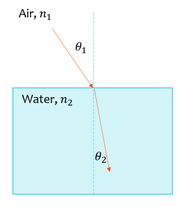# Top Physics Formulas

Here you will find the most common physics equations and formulas used in high school and fundamental university courses. These include, but are not limited to, mechanics, kinematics, energy, uniform circular motion, and waves formulas.

For more physics formulas visit Wikipedia.

## Basic Mechanics Equations

### Velocity

=
 Δx Δt
v  =
 dx dt

Where x is the displacement and t the time. The first equation gives the average velocity while the second equation is the instantaneous velocity

### Acceleration

=
 Δv Δt
a  =
 dv dt

Where v is the velocity and t the time. The first equation gives the average acceleration while the second equation is the instantaneous acceleration

### Force

 F = ma

Where m is the mass of the object and a is the acceleration

### Weight

 w = mg

Where m is the mass and g is the gravitational acceleration. On earth, it is 9.8 m/s2.

## Kinematics Equations (Equations of Motion)

1. v  =  v0 + at
2. Δx  =
 v + v0 2
t
3. Δx  =   v0t   +
 1 2
at2
4.  v2  =  v02  +  2aΔx
 Δx Displacement t Time v0 Initial Velocity v Final Velocity a Acceleration

• To be used under constant acceleration

## Energy

### Gravitational Potential Energy

 PEg  =  mgh [Joules]Where m is the mass of the object, g is the gravitational constant 9.8 m/s2, and h is the height of the object

### Kinetic Energy

KE  =
 1 2
mv2 [Joules]

Where m is the mass of the object, v is the velocity of the object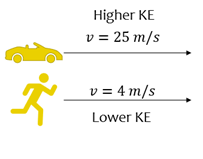### Elastic Potential Energy

PEx  =
 1 2
kx2 [Joules]

Where k is the spring constant and x is the displacement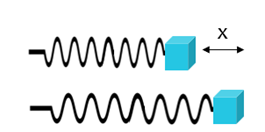## Uniform Circular Motion

### Angular Velocity & Acceleration

ω̄  =
 Δθ Δt
ω  =
 dθ dt
ᾱ  =
 Δω Δt
α  =
 dω dt

Where theta is the angular displacement. The first equation gives the average angular velocity, while the second is the instantaneous angular velocity. The third equation gives the average angular acceleration, while the fourth is the instantaneous angular acceleration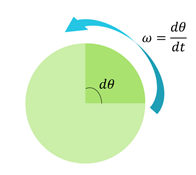### Linear Velocity & Acceleration

 v  =  ωr

 a  =  αxr - ω2r

The angular velocity ω can be converted into a linear velocity v with this equation where r is the radius.
The angular acceleration α can be converted into a linear acceleration a with this equation where r is the radius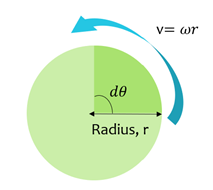### Torque

 τ  =  r x F

Where F is the force acting in the direction of rotation, and r is the arm length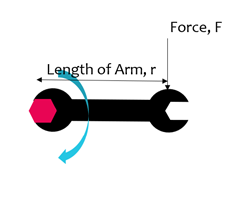## Waves & Light

### Frequency of EM Wave

ƒ  =
 1 T

Where T is the period of the wave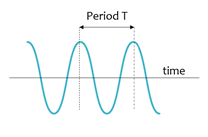### Velocity of EM Wave

 v  = λƒ

Where λ is the wavelength and ƒ is the frequency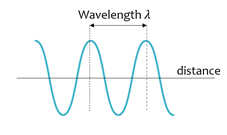### Snell’s Law

 n1θ  =   n2θ

Where n1 and n2 are the indices of refraction for the given mediums and θ1 is the angle of incidence and θ2 is the angle of refraction